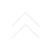#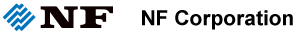Driving the future of technology : Original solution in measurement and control

## Lecture for measurement

2020/5/20 posted

### Measurement of Small AC Signals and Low Noise Superimposed DC Signal

#### 2. Measurement Examples

(1) Measurement of small sine-wave (100 µVp-p, 100 kHz) superimposed on 8.5 V of DC signal

A test signal which is a sine wave of 100 µVp-p, 100 kHz superimposed on 8.5 V of DC voltage, was measured in two different ways as below. (Figure 3.)
• a) Measurement via a digital oscilloscope only
• b) Measurement via a digital oscilloscope and NF developed pre-amplifier

• With a digital oscilloscope only, the superimposed sine wave cannot be observed, yet though the NF developed pre-amplifier, the observation of the sine wave 100 µVp-p, 100 kHz superimposed on an 8.5 V of DC voltage has become possible.

Figure 3. Measurement of superimposed sine wave of 100 µVp-p, 100 kHz on 8.5 V of DC voltage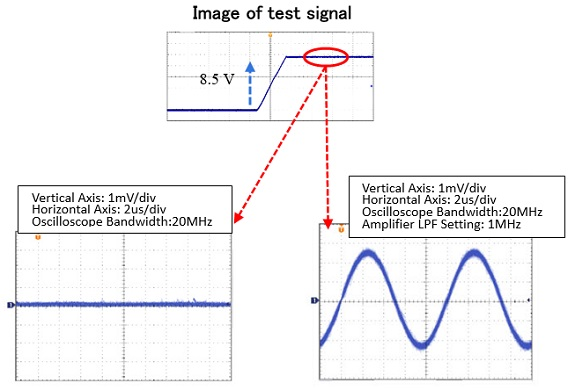a) Superimposed signal waveform measured with a digital oscilloscope only b) Superimposed signal waveform measured with NF pre-amplifier + digital oscilloscope

(2) Measurement of output noise waveform of linear power supply

Measured output noise waveform of a low noise linear power supply is as shown below. (Figure 4.) Most of the noise is humming noise, however we can see that very large and instantaneous noise is generated in the hum cycle.

Figure 4. Measurement of noise superimposed on output of linear power supply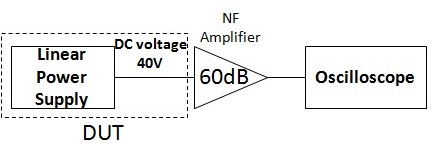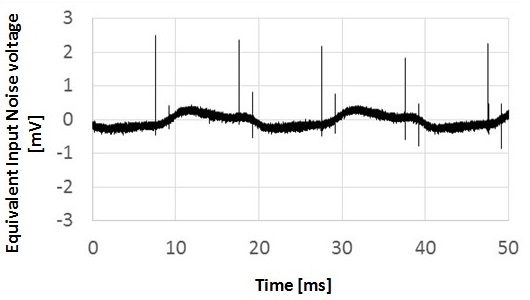Block diagram of the Measuring System Output noise waveform of linear power supply measured with NF pre-amplifier + digital oscilloscope

(3) Measurement of output noise waveform of DC/DC converter

Measured output noise waveform of a DC to DC converter with 5 V output is shown as below. (Figure 5.) We can see that the noise caused by switching is dominant.

Figure 5. Measurement of noise waveform superimposed on a 5 V output of DC-DC converter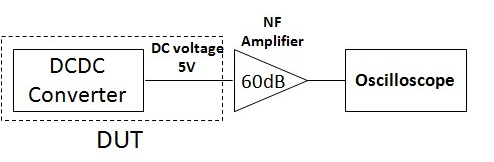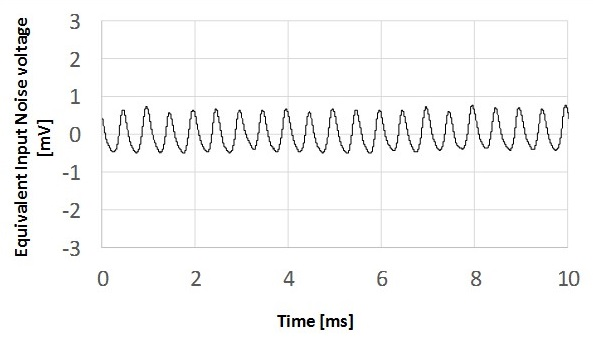Block diagram of measuring system Output noise waveform of DC/DC converter measured with NF pre-amplifier + digital oscilloscope

(4) Measurement of output noise waveform of high voltage amplifier

The output of low noise DC power supply is amplified by a high voltage amplifier, and measurement data of the noise waveform superimposed on high voltage of 40 V DC is shown as below. (Figure 6.)
As we can see, about 1 mV of noise superimposed on a 40 V signal can be detected, and white noise is shown to be dominant.

Figure 6. Measurement of noise waveform superimposed on 40 V of DC voltage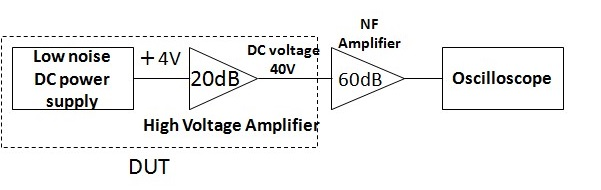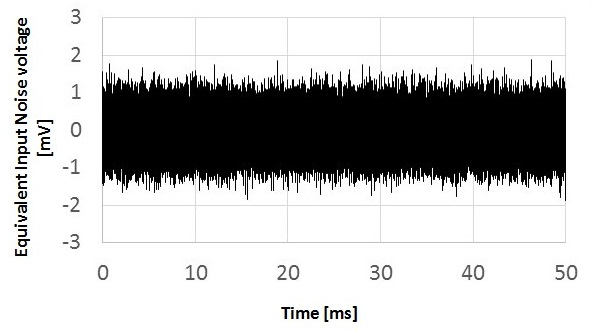Block diagram of measuring system Noise waveform of high voltage amplifier output measured with NF pre-amplifier + digital oscilloscope

#### 3. Applications

•  Observation and evaluation of power supply output noise
•  Voltage measurement of power from solar cells
•  Observation and evaluation of DC/DC converter output noise

Recent post